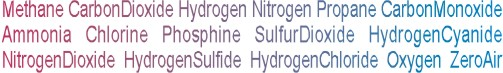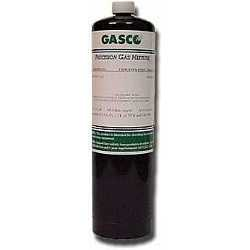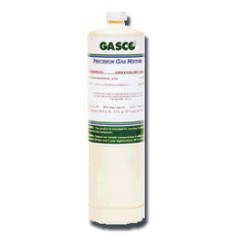Calibration gas blends, mixes and accessories
NON-REACTIVE SINGLE GAS > OXYGEN :    41 Items found.(1515)

NORLAB Equivalent  P107219

Norlab Equivalent Calibration Gas 19.0% Oxygen Balance nitrogen 17L Liter cylinder (Made by Gasco)

Configure

\$36.47(1506)

NORLAB Equivalent  P107204

Norlab Equivalent Calibration Gas 0.4% Oxygen Balance nitrogen 17L Liter cylinder (Made by Gasco)

Configure

\$36.47(1516)

NORLAB Equivalent  P1072195

Norlab Equivalent Calibration Gas 19.5% Oxygen Balance nitrogen 17L Liter cylinder (Made by Gasco)

Configure

\$36.47(1514)

NORLAB Equivalent  P107218

Norlab Equivalent Calibration Gas 18.0% Oxygen Balance nitrogen 17L Liter cylinder (Made by Gasco)

Configure

\$36.47(1513)

NORLAB Equivalent  P107217

Norlab Equivalent Calibration Gas 17.0% Oxygen Balance nitrogen 17L Liter cylinder (Made by Gasco)

Configure

\$36.47(1512)

NORLAB Equivalent  P107215

Norlab Equivalent Calibration Gas 15.0% Oxygen Balance nitrogen 17L Liter cylinder (Made by Gasco)

Configure

\$36.47(1511)

NORLAB Equivalent  P10728

Norlab Equivalent Calibration Gas 8.0% Oxygen Balance nitrogen 17L Liter cylinder (Made by Gasco)

Configure

\$36.47(1510)

NORLAB Equivalent  P10727

Norlab Equivalent Calibration Gas 7.0% Oxygen Balance nitrogen 17L Liter cylinder (Made by Gasco)

Configure

\$36.47(1509)

NORLAB Equivalent  P10725

Norlab Equivalent Calibration Gas 5.0% Oxygen Balance nitrogen 17L Liter cylinder (Made by Gasco)

Configure

\$36.47(1508)

NORLAB Equivalent  P10724

Norlab Equivalent Calibration Gas 4.0% Oxygen Balance nitrogen 17L Liter cylinder (Made by Gasco)

Configure

\$36.47(1507)

NORLAB Equivalent  P10722

Norlab Equivalent Calibration Gas 2.0% Oxygen Balance nitrogen 17L Liter cylinder (Made by Gasco)

Configure

\$36.47(1517)

NORLAB Equivalent  P1072209

Norlab Equivalent Calibration Gas 20.9% Oxygen Balance nitrogen 17L Liter cylinder (Made by Gasco)

Configure

\$36.47(1527)

NORLAB Equivalent  HP107219

Norlab Equivalent Calibration Gas 19.0% Oxygen Balance nitrogen 34LS Liter cylinder (Made by Gasco)

Configure

\$51.53(1518)

NORLAB Equivalent  HP107204

Norlab Equivalent Calibration Gas 0.4% Oxygen Balance nitrogen 34LS Liter cylinder (Made by Gasco)

Configure

\$51.53(1519)

NORLAB Equivalent  HP10722

Norlab Equivalent Calibration Gas 2.0% Oxygen Balance nitrogen 34LS Liter cylinder (Made by Gasco)

Configure

\$51.53(1520)

NORLAB Equivalent  HP10724

Norlab Equivalent Calibration Gas 4.0% Oxygen Balance nitrogen 34LS Liter cylinder (Made by Gasco)

Configure

\$51.53(1521)

NORLAB Equivalent  HP10725

Norlab Equivalent Calibration Gas 5.0% Oxygen Balance nitrogen 34LS Liter cylinder (Made by Gasco)

Configure

\$51.53(1522)

NORLAB Equivalent  HP10727

Norlab Equivalent Calibration Gas 7.0% Oxygen Balance nitrogen 34LS Liter cylinder (Made by Gasco)

Configure

\$51.53(1523)

NORLAB Equivalent  HP10728

Norlab Equivalent Calibration Gas 8.0% Oxygen Balance nitrogen 34LS Liter cylinder (Made by Gasco)

Configure

\$51.53(1524)

NORLAB Equivalent  HP107215

Norlab Equivalent Calibration Gas 15.0% Oxygen Balance nitrogen 34LS Liter cylinder (Made by Gasco)

Configure

\$51.53
Page: *1 | 2 | 3 | All